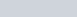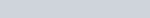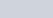Remember:

• f(|x|) reflects the graph to the right of the y-axis in the y-axis. Ignore the left hand side part of the graph

In this video I show you how to draw graphs of the form y=f(|x|) using the modulus function and give you three graphs to try.

Examples in the video: Sketch the following

1.2.3.Remember: f(|x|) reflects the graph to the right of the y-axis in the y-axis. Ignore the left hand side part of the graph In this video I show you how to draw graphs of the form y=f(|x|) using the modulus function and give you three graphs to try. Examples in the video: Sketch the following [latex]y